# Texas Go Math Grade 8 Lesson 5.2 Answer Key Writing Linear Equations from a Table

Refer to our Texas Go Math Grade 8 Answer Key Pdf to score good marks in the exams. Test yourself by practicing the problems from Texas Go Math Grade 8 Lesson 5.2 Answer Key Writing Linear Equations from a Table.

## Texas Go Math Grade 8 Lesson 5.2 Answer Key Writing Linear Equations from a Table

Essential Question
How do you write an equation to model a linear relationship given a table?

Question 1.
The table shows the volume of water released by Hoover Dam over a certain period of time. Graph the data, and find the slope and y-intercept from the graph. Then write the equation for the graph in slope-intercept form.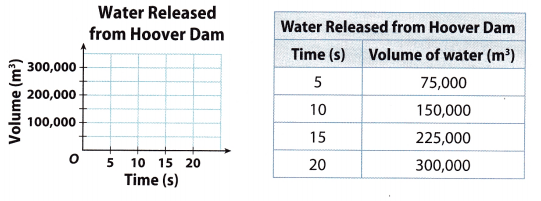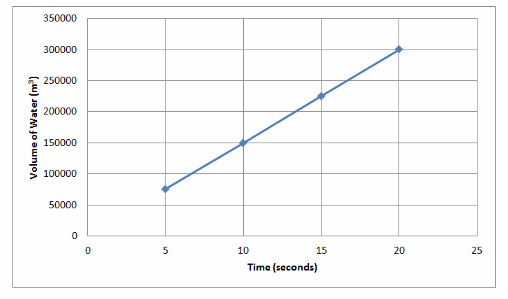Graph the ordered pairs from the table and draw a line through the points.
Slope = $$\frac{150000-75000}{10-5}$$ = $$\frac{75000}{5}$$ = 15000
Find the slope using two points on the graph by
Slope(m) = $$\frac{y_{2}-y_{1}}{x_{2}-x_{1}}$$
where
(x2, y2) = (10, 150000) and (x1, y1) = (5, 75000)
b = 0 Read the y-intercept from the graph
y = 15000x Substituting the value of slope (m) and y intercept in y = mx + b

Example 2.
Elizabeth’s cell phone plan lets her choose how many minutes are included each month. The table shows the plan’s monthly cost y for a given number of included minutes x. Write an equation in slope-intercept form to represent the situation.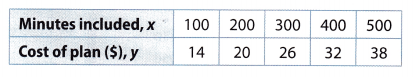Step 1
Notice that the change in cost is the same for each increase of 100 minutes. So, the relationship is linear. Choose any two ordered pairs from the table to find the slope.Step 2
Find the y-intercept. Use the slope and any point from the table.Step 3’
Substitute the slope and y-intercept.
y = mx + b Slope-intercept form
y = 0.06x + 8 Substitute 0.06 for m and 8 for b.

Reflect

Math Grade 8 Answer Key Pdf Lesson 5.2 Using Intercepts Question 2.
What is the base price for the cell phone plan, regardless of how many minutes are included? What is the cost per minute? Explain.
y = 0.06x + 8 From the example 2
The base price = $8 It is the y-intercept, the cost of plan when 0 minutes are included Cost per minute =$0.06 It is the slope, the cost of call per minute

Question 3.
What If? Elizabeth’s cell phone company changes the cost of her plan as shown below. Write an equation in slope-intercept form to represent the situation. How did the plan change?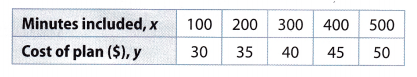Slope = $$\frac{35-30}{200-100}$$ = $$\frac{5}{100}$$ = 0.05
Find the slope using two points on from the table by
Slope(m) = $$\frac{y_{2}-y_{1}}{x_{2}-x_{1}}$$
where
(x2, y2) = (200, 35)) and
(x1, y1) = (100, 30)

30 = 0.05(100) + b Substituting the value of slope (m) and (x, y) in the slope-intercept form to find y-intercept (b):
30 = 5 + b y = mx + b
b = 30 – 5 = 25

y = 0.05x+25 . Substituting the value of slope (m) and y-intercept in slope-intercept form.
y = mx + b
The base price increases to $\$25$and the cost per minute decreases to$\$0.05$ per minute.

Question 4.
A salesperson receives a weekly salary plus a commission for each computer sold. The table shows the total pay, p, and the number of computers sold, n. Write an equation in slope-intercept form to represent this situation.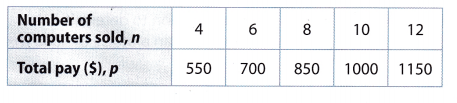Slope = $$\frac{700-550}{6-4}$$ = $$\frac{150}{2}$$ = 75
Find the slope using two points from the table by
where
(x2, y2) = (6, 700) and (x1, y1) = (4, 550)

700 = 75(6) + b Substituting the value of slope (m) and (x, y) in the slope-intercept form to find y-intercept (b):
700 = 450 + b y = mx + b
b = 700 – 450 = 250
p = 75n + 250 Substituting the value of slope (m) and y-intercept in slope-intercept form. y = mx + b
Note that p is represented by the axis and n is represented by the axis

Lesson 5.2 Writing Linear Equations from a Table Answer Key Question 5.
To rent a van, a moving company charges a daily fee plus a fee per mile. The table shows the total cost, c, and the number of miles driven, d. Write an equation in slope-intercept form to represent this situation.Slope = $$\frac{50-45}{20-10}$$ = $$\frac{5}{10}$$ = 0.5
Find the slope using two points on from the table by
Slope(m) = $$\frac{y_{2}-y_{1}}{x_{2}-x_{1}}$$
where
(x2, y2) (20, 50) and (x1, y1) = (10, 45)
50 = 0.5(20) + b Substituting the value of slope (m) and (x, y) in the slope-intercept form to find y-intercept (b): y = mx + b
b = 50 – 10 = 40

c = 0.5d + 40 Substituting the value of slope (m) and y-intercept in slope-intercept form. y = mx + b
Note that c is represented by the axis and d is represented by the axis

Question 1.
Jaime purchased a $20 bus pass. Each time he rides the bus, a certain amount is deducted from the pass. The table shows the amount, y, left on his pass after x rides. Graph the data, and find the slope and y-intercept from the graph. Then write the equation for the graph in slope-intercept form. (Example 1)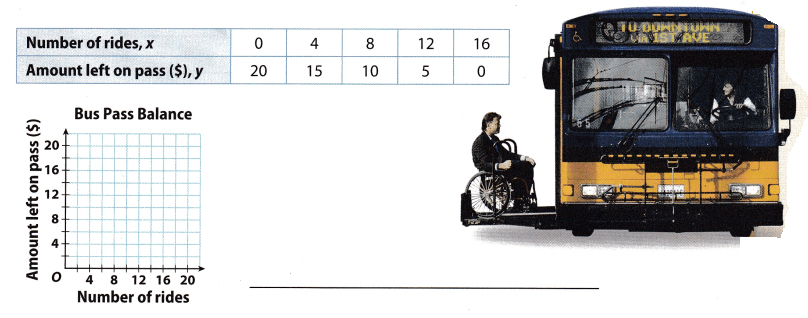Answer: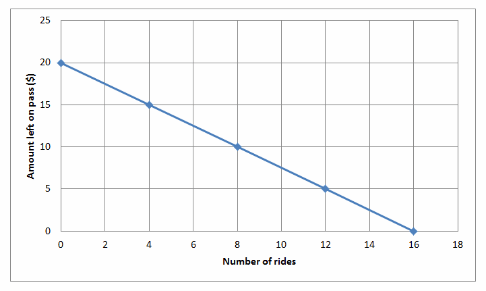Graph the ordered pairs from the table and draw a line through the points. Slope(m) = $$\frac{20-15}{0-4}$$ = $$\frac{5}{-4}$$ = -1.25 Find the slope using two points on the graph by where slope(m) = $$\frac{y_{2}-y_{1}}{x_{2}-x_{1}}$$ where (x2, y2) = (4, 15) and (x1, y1) = (0, 20) b = 20 Read the y-intercept from the graph y = -2.5x + 20 Substituting the value of slope (m) and y-intercept in slope-intercept form. y = mx + b The table shows the temperature (y) at different altitudes (x). This is a linear relationship. (Example 2)Writing Equations from a Table Worksheet Answer Key Question 2. Find the slope for this relationship. Answer: From the table we can see that the rate of change is constant, so we can use the ratio $$\frac{y_{2}-y_{1}}{x_{2}-x_{1}}$$ to find the slope. The relationship is linear. Choose two points from the table and put them in the calculation.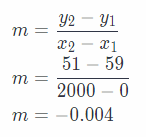Question 3. Find the y-intercept for this relationship. Answer: b = 9 Read the y-intercept from the table (when x = 0) Question 4. Write an equation in slope-intercept form that represents this relationship. Answer: y = -0.004x + 59 Substituting the value of slope (m) and y intercept in slope-intercept form. y = mx + b Question 5. Use your equation to determine the temperature at an altitude of 5,000 feet. Answer: The equation for the given table is y = -0.004x + 59. We need to determine the temperature (y), measured in degrees Fahrenheit, at an altitude of 5000 feet (x). Put the given value of x into the equation. y = -0.004 . 5000 + 59 y = -20 + 59 y = 39 So the temperature at an altitude of 5000 feet is 39 degrees Fahrenheit. Essential Question Check-In Answer Key Math Grade 8 Lesson 5.2 Writing Linear Equations from a Table Question 6. Describe how you can use the information in a table showing a linear relationship to find the slope and y-intercept for the equation. Answer: Use any two points from the table to find the slope using, Slope(m) = $$\frac{y_{2}-y_{1}}{x_{2}-x_{1}}$$ If the value of y-intercept, when x = 0 is not given in the table, use the slope and any ordered pair from the table and substitute in slope-intercept form: y = mx + b to find b. Texas Go Math Grade 8 Lesson 5.2 Independent Practice Answer Key Question 7. The table shows the costs of a large cheese pizza with toppings at a local pizzeria. Graph the data, and find the slope and y-intercept from the graph. Then write the equation for the graph in slope-intercept form.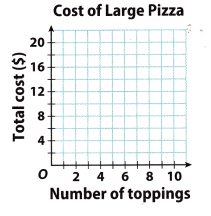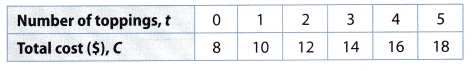Answer: Plot the given values of x and y on the graphFrom the table we can see that the rate of change is constant, so we can use the ratio $$\frac{y_{2}-y_{1}}{x_{2}-x_{1}}$$ to find the slope. The relationship is linear. Choose two points from the table and put it in the calculation, m = $$\frac{y_{2}-y_{1}}{x_{2}-x_{1}}$$ m = $$\frac{12-10}{2-1}$$ m = $$\frac{2}{1}$$ m = 2 The starting point on the graph is (0, 8), i.e. (0, b). So the value of the y-intercept is 8. → b = 8 The standard form of the linear equation is y = mx + b, so include the obtained values for the slope and y-intercept: y = 2x + 8 Question 8. The table shows how much an air-conditioning repair company charges for different numbers of hours of work. Graph the data, and find the slope and y-intercept from the graph. Then write the equation for the graph in slope-intercept form.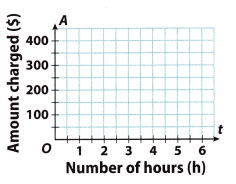Answer: plot the given values of x and y on the graph.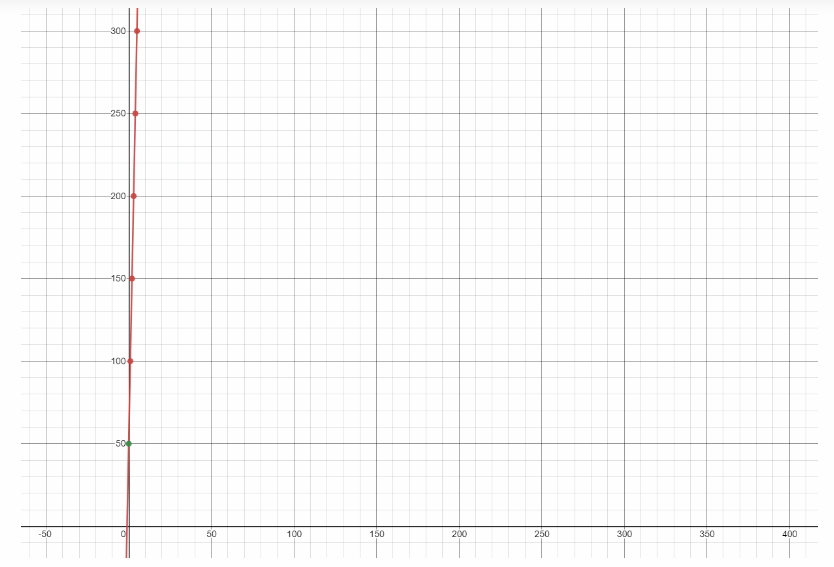From the table we can see that the rate of change is constant, so we can use the ratio $$\frac{y_{2}-y_{1}}{x_{2}-x_{1}}$$ to find the slope. The relationship is linear. Choose two points from the table and put it in the calculation. m = $$\frac{y_{2}-y_{1}}{x_{2}-x_{1}}$$ m = $$\frac{150-100}{2-1}$$ m = $$\frac{50}{1}$$ m = 50 The starting point on the graph is (0, 50), i.e. (0, b). So the value of the y-intercept is 50. → b = 50 The standard form of the linear equation is y = mx + b, so include the obtained values for the slope and y-intercept: y = 50x + 50 Go Math 8th Grade Pdf Lesson 5.2 Answer Key Question 9. A friend gave Ms. Morris a gift card for a number of car local car wash. The table shows the linear relationship of how the value left on the Amount left on the card relates to the number of car washes.a. Write an equation that shows the number of dollars left on the card. Answer: Slope = $$\frac{18-30}{8-0}$$ = $$\frac{-12}{8}$$ = -1.5 Find the slope using two points from the table by Slope(m) = $$\frac{y_{2}-y_{1}}{x_{2}-x_{1}}$$ where (x2, y2) = (8, 18) and (x1, y1) = (0, 30) b = 30 Read the y-intercept from the table (when x = 0) y = -1.50x + 30 Substituting the value of slope (m) and y-intercept in slope-intercept form. y = mx + b b. Explain the meaning of the negative slope in this situation. Answer: The negative slope means that the amount on the card is decreased by$1.5 per car wash. Meaning of negative slope

c. What is the maximum value of x that makes sense in this context? Explain.
0 = -1.50x + 30 The maximum value of s that makes sense in this context is when $0 is left on the card i.e. y = 0 1.5x = 30 x = $$\frac{30}{1.5}$$ = 20 The maximum value of$x = 20$The tables show linear relationships between x and y. Write an equation in slope-intercept form for each relationship. Question 10.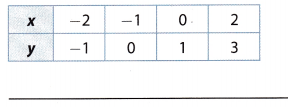Answer: The slope is calculated by: m = $$\frac{y_{2}-y_{1}}{x_{2}-x_{1}}$$ Put the given values of x and y from the table into the calculation. Choose two points. m = $$\frac{0-(-1)}{-1-(-2)}$$ m = $$\frac{0+1}{-1+2}$$ m = $$\frac{1}{1}$$ m = 1 The standard form of the linear equation is y = mx + b, so include the obtained values of x, y, and the slope to get y-intercept. For x and y, choose one point from the table. y = mx + b -1 = 1 • (-2) + b -1 = -2 + b -1 + 2 = b b = 1 Equation in slope-intercept form is: y = 1x + 1 → y = x + 1 Writing Equations from a Table Worksheet Answer Key Pdf Question 11.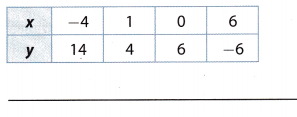Answer: The slope is calculated by: m = $$\frac{y_{2}-y_{1}}{x_{2}-x_{1}}$$ Put the given values of x and y from the table into the calculation. Choose two points. m = $$\frac{4-14}{1-(-4)}$$ m = $$\frac{-10}{1+4}$$ m = $$\frac{-10}{5}$$ m = $$-\frac{10}{5}$$ m = -2 The standard form of the linear equation is y = mx + b, so include the obtained values of x, y, and the slope to get y-intercept. For x and y, choose one point from the table. y = mx + b 4 = -2 • 1 + b 4 = -2 + b 4 + 2 = b b = 6 The equation in slope-intercept form is: y = 2x + 6 Question 12. Finance Desiree starts a savings account with$125.00. Every month, she deposits $53.50. a. Complete the table to model the situation.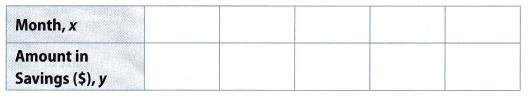Answer:complete the table b. Write an equation in slope-intercept form that shows how much money Desiree has in her savings account after x months. Answer: y = 53.5x + 125 Substituting the value of slope (m) and y-intercept in slope-intercept form. y = mx + b where, m = 53.5 and b = 125 c. Use the equation to find how much money Desiree will have in savings after 11 months. Answer: y = 53.5(11) + 123 Substitute x = 11 months y = 588.5 + 125 = 713.5 Desiree will have$713.5 after 11 months

Monty documented the amount of rain his farm received on a monthly basis, as shown in the table.a. Is the relationship linear? Why or why not?
No
The change in the months is constant but the change in rainfall is not constant

b. Can an equation be written to describe the amount of rain? Explain.
No
There is no apparent pattern in the given data.

Texas Go Math Grade 8 Lesson 5.2 H.O.T. Focus On Higher Order Thinking Answer Key

Question 14.
Analyze Relationships If you have a table that shows a linear relationship, when can you read the value for b, in y = mx+ b, directly from the table without drawing a graph or doing any calculations? Explain.